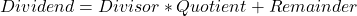One of the types of math questions you will see on the GRE exam are questions that require the use of basic division. This video shows how to use it to solve the following example GRE exam problem:

When the integer k is divided by 18, the quotient is x and the remainder is 5. When integer k is divided by 29, the quotient is y and the remainder is 13. Which of the following equations is true?

a. 18x+29y=8

b. 18x-29y=8

c. 18x+29y=-8

d. 18x-29y=-8

Approach: This is a pretty much straightforward question where we need to apply the relationSo, k=18x+5 and k=29y+13.

Here’s the video solution: